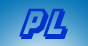Solving Diophantine Equations Home  | Send Your Feedback

Diophantine Equations are those in which there are more
variables than equations and hence multiple solutions.
Usually, integer solutions are required.

This page finds integer solutions to single linear
Diophantine Equations of the form
• A x + B y = C
where A, B, and C are given.

Since A(x + n*B) + B(y - n*A) = Ax + By + ABn - ABn,
given one solution, you can add or subtract any multiple of B to x, while doing the opposite (subtracting or adding) with the same multiple of A to y.

Example: "Solve 7x + 13y = 102"

Starting from x = 9, y = 3 (which gives 63 + 39 = 102)
other solutions are:
 N 9 + N*13 3 - N*7 7 x + 13 y = 102 -2 9-26 = -17 3+14=17 7*(-17) + 13*17 = -119 + 221 = 102 -1 9-13 = -4 3+7=10 7*(-4) + 13*10 = -28 + 130 =102 1 9+13 = 22 3-7=-4 7*22 - 13*4 = 154-52 = 102 2 9+26 = 35 3-14=-11 7*35 - 13*11 = 245-143 = 102

To solve a Diophantine Equation,
enter the three integers you want as coefficients,
and press "Solve".

 Ax + By = C A = * x + B = * y = C =

The Old Water Jug Problem
explains the solution method used.